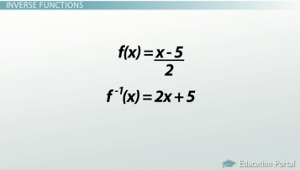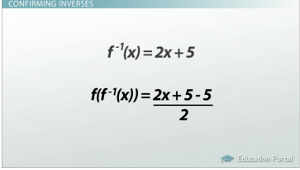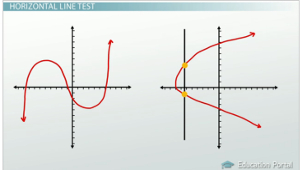# Inverse Functions

An error occurred trying to load this video.

Try refreshing the page, or contact customer support.

Coming up next: Applying Function Operations Practice Problems

### You're on a roll. Keep up the good work!

Replay
Your next lesson will play in 10 seconds
• 0:05 Inverse Functions
• 1:33 Confirming Inverses
• 2:46 Finding Inverses
• 4:00 Horizontal Line Test
• 5:18 Lesson Summary

Want to watch this again later?

Timeline
Autoplay
Autoplay
Speed

#### Recommended Lessons and Courses for You

Lesson Transcript
Instructor: Luke Winspur

Luke has taught high school algebra and geometry, college calculus, and has a master's degree in education.

Inverse functions are two functions that do exactly opposite things. Check out this lesson to learn about how to write inverse functions, find inverse functions, and predict whether or not they exist.

## Inverse FunctionsYou probably haven't had to watch very many of these videos to hear me say the words 'inverse operations.' I use this term to talk about how we can solve algebraic equations - maybe like this one: 2x + 3 = 9 - by undoing each number around the variable. For example, we undo a plus 3 with a minus 3 because addition and subtraction are inverse operations. We can then also undo a times by 2 with a divide by 2, again, because multiplication and division are inverse operations.

In just the same way, an inverse function is a function that is the opposite of another one. If a function is a set of steps to do to a number, its inverse is just a different set of steps that undoes everything the original function did.

For example, if we had one function f(x) that told us to start with a number, subtract 5, and then divide by 2, its inverse function would, instead, do all the opposite operations in the opposite order. That means that it would start with the same number, multiply it by 2 first, and then add 5.

Notice that we use this f to the -1 power notation to indicate an inverse function. This has to do with what an exponent of -1 means, but it's not really important that you understand why we use it, only that we do.

## Confirming InversesOnce we have a function and its inverse, we can check to make sure they are inverses by using function composition. If you're not familiar with this process, there is a video you can watch to learn about it, but basically it means plugging one function into another. The idea is that since inverse functions do the exact opposite things, if we compose them together, we should end up exactly where we started - just a plain x. Let's try this with our example above.

Substituting in f-1 into f means evaluating f(f-1 (x)). Plugging an f inverse function wherever we used to see an x in the f function makes our equation look like this: f(f-1 (x)) = (2x + 5 - 5) / 2. Right away we see the different steps canceling out. The +5 and the -5 in the numerator undo each other, leaving us here: f(f-1 (x)) = 2x / 2, and then the times 2 and divided by 2 undo to bring us right back to where we started - plain old x. This process actually shows us the mathematical definition of inverse functions: two functions that, when composed with each other, cancel out and get us right back to the x on the inside.

## Finding Inverses

So, now that we know what inverse functions are, how might you go about finding one? Say we have the function g(x) = √(2x - 3) shown here, what would the inverse of g be?Well, okay, since g(x) is really just a different way of saying y, we could rewrite our function like this: y = √(2x - 3). Now, relying on the knowledge that the inverse is a list of steps that are the exact opposite as the original, we can use the following trick to find any inverse function. Switching the x and the y and now solving the equation for y with inverse operations will provide us with the exact set of steps that will undo the original function.

To unlock this lesson you must be a Study.com Member.

### Register to view this lesson

Are you a student or a teacher?

### Unlock Your Education

#### See for yourself why 30 million people use Study.com

##### Become a Study.com member and start learning now.
Back
What teachers are saying about Study.com

### Earning College Credit

Did you know… We have over 160 college courses that prepare you to earn credit by exam that is accepted by over 1,500 colleges and universities. You can test out of the first two years of college and save thousands off your degree. Anyone can earn credit-by-exam regardless of age or education level.